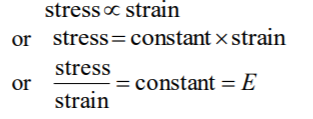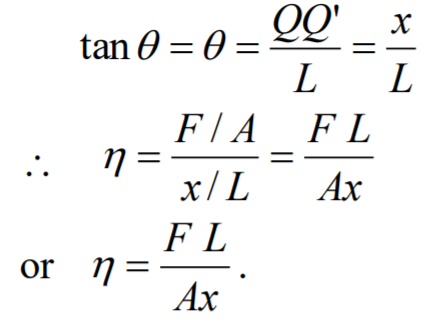Elastic ModulusHooke's Law (In terms of Stress and Strain)

↪ Young modified the original form of Hooke's law which states that within elastic limit, stress is directly proportional to strain. That is,where E is called the modulus of elasticity.
There are different types of modulus of elasticity.

Types of Moduli of Elasticity

﻿Corresponding to three types of stress and strain there are three types of modulus of elasticity.
﻿They are described below:

﻿Young's Modulus of Elasticity↪ The Young's modulus of an elastic wire is defined as the ratio of the tensile stress to the tensile strain and is denoted by Y↪ Suppose a uniform wire of length L and cross-section of area A is fixed at one end as shown in the figure.
↪ Suppose a force F is applied to the free end to pull down the wire and its length increases by an extension or elongation l.↪ If the wire has cross-section of radius r, thenApplying different forces F at the free end, the corresponding extension l can be determined and the value of Y can be calculated experimentally.

Bulk Modulus of Elasticity↪ The bulk modulus of elasticity of an object is defined as the ratio of the excess pressure to the volume strain.
↪ Pressure is a particular kind of stress which changes in the volume of the object.
↪ A small increase in pressure ∆p applied to the object decreases in its volume from V to V – ∆V so that ∆V is a small decrease in volume.
↪ Thus from the definition, the bulk modulus B is↪ The negative sign shows that the volume decreases as the pressure increases. The reciprocal of the bulk modulus is called the compressibility and is denoted by K. That is,Modulus of Rigidity↪ The modulus of rigidity of an object is defined as the ratio of the tangential stress to the shear strain. It is denoted by ɳ. So the modulus of rigidity is↪ Let us consider a rectangular block whose lower face MNOP is fixed and upper face QRST is subjected to a tangential face F.
↪ Let A be the area of the face QRST.
↪ The upper face at a distance L from the lower face has been displaced through a distance QQ' = x so that the shear strain is